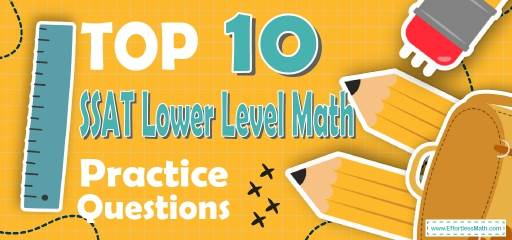# Top 10 SSAT Lower Level Math Practice QuestionsThe best way to prepare your students for the SSAT Lower Level Math test is to work through as many SSAT Lower Level Math practice questions as possible. Here are the top 10 SSAT Lower Level Math practice questions to help your students review the most important SSAT Lower Level Math concepts. These SSAT Lower Level Math practice questions are designed to cover mathematics concepts and topics that are found on the actual test. The questions have been fully updated to reflect the latest 2022 SSAT Lower Level guidelines. Answers and full explanations are provided at the end of the post.

Help your students start their SSAT Lower Level Math test prep journey right now with these samples SSAT Lower Level Math questions.

## SSAT Lower Level Math Practice Questions

1- Which of the following statement is False?

A. $$2×2=4$$

B. $$(4+1)×5=25$$

C. $$6÷(3−1)=1$$

D. $$6×(4−2)=12$$

E.$$(10+23)×10=330$$

2- If all the sides in the following figure are of equal length and the length of one side is $$4$$, what is the perimeter of the figure?

A. $$15$$

B. $$18$$

C. $$20$$

D. $$24$$

E. $$28$$

3- $$\frac{4}{5}\ −\ \frac{3}{5}$$= ?

A. $$0.3$$

B. $$0.35$$

C. $$0.2$$

D. $$0.25$$

E. $$0.1$$

4-If $$?=2$$ and $$\frac{64}{?}+4= ☐$$, then $$☐= …..$$

A. $$30$$

B. $$32$$

C. $$34$$

D. $$36$$

E. $$38$$

5- If (?) is an even number, which of the following is always an odd number?

A. $$\frac{N}{2}$$

B. $$N+4$$

C. $$2N$$

D. $$(2×?)+2$$

E. $$N+1$$

6- In the following figure, the shaded squares are what fractional part of the whole set of squares?

A. $$\frac{1}{2}$$

B. $$\frac{5}{8}$$

C. $$\frac{2}{3}$$

D. $$\frac{3}{4}$$

E. $$\frac{5}{6}$$

7- Which of the following is greater than $$\frac{3}{2}$$?

A. $$\frac{1}{2}$$

B. $$\frac{5}{2}$$

C. $$\frac{3}{4}$$

D. $$1$$

E. $$1.4$$

8- If $$\frac{1}{3}$$ of a number is greater than $$6$$, the number must be …..

A. Less than $$3$$

B. Equal to $$3$$

C. Equal to $$18$$

D. Greater than $$18$$

E. Equal to $$6$$

9- If $$4×(?+?)=20$$ and $$M$$ is greater than $$0$$, then $$N$$ could Not be …..

A. $$1$$

B. $$2$$

C. $$3$$

D. $$4$$

E. $$5$$

10- Which of the following is closest to $$5.03$$?

A. $$6$$

B. $$5.5$$

C. $$5$$

D. $$5.4$$

E. $$6.5$$

## Best SSAT Lower Level Math Exercise Resource for 2022

1- C
A)$$2×2=4$$ This is true!
B)$$(4+1)×5=25$$ This is true!
C)$$6÷(3-1)=1→6÷2=3$$ This is NOT true!
D)$$6×(4-2)=12→6×2=12$$ This is true!
E)$$(10+23)×10=330→33×10=330$$ This is true!

2- D
The shape has $$6$$ equal sides. And is side is $$4$$. Then, the perimeter of the shape is: $$4×6=24$$

3- C
$$\frac{4}{5}-\frac{3}{5}=\frac{1}{5}=0.2$$

4- D
$$N=2$$ , then: $$\frac{64}{2}+4=32+4=36$$

5- E
N is even. Let’s choose $$2$$ and $$4$$ for $$N$$. Now, let’s review the options provided.
A. $$\frac{N}{2}=\frac{2}{2}=1, \frac{N}{2}=\frac{4}{2}=2$$, One result is odd and the other one is even.
B. $$N+4=2+4=6,4+4=8$$
Both results are even.
C. $$2N=2×2=4,4×2=8$$
Both results are even.
D. $$(2×N)+2=(2×2)+2=6,(4×2)+2=10$$
Both results are even.
E. $$N+1=2+1=3,4+1=5$$
Both results are odd.

6- A
There are $$10$$ squares and $$5$$ of them are shaded. Therefore, $$5$$ out of $$10$$ or $$\frac{5}{10}=\frac{1}{2}$$ are shaded.

7- B
$$\frac{3}{2}=1.5$$, The only option that is greater than $$1.5$$ is $$\frac{5}{2}$$
$$\frac{5}{2}=2.5 \ , \ 2.5>1.5$$

8- D
If $$\frac{1}{3}$$ of a number is greater than $$6$$, the number must be greater than $$18$$.
$$\frac{1}{3} x>6→x>18$$

9- E
$$4×(M+N)=20$$, then $$M+N=5. M>0→N$$ could not be $$5$$.

10- C
The closest to $$5.03$$ is $$5$$ in the options provided.

Looking for the best resource to help you succeed on the SSAT Lower Level Math test?

## The Best Books to Ace the SSAT Lower Level Math Test

### What people say about "Top 10 SSAT Lower Level Math Practice Questions - Effortless Math: We Help Students Learn to LOVE Mathematics"?

No one replied yet.

X
30% OFF

Limited time only!

Save Over 30%

SAVE $5 It was$16.99 now it is \$11.99